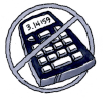### Home > MC1 > Chapter 9 > Lesson 9.2.5 > Problem9-107

9-107.

Autumn and her friends had dinner at their favorite restaurant, and the bill came to \$60. They decided to leave 18% of the bill for a tip. Without using a calculator, help them compute how much money is 18% of the bill.

$10\%$ of $60$ is $6$
$5\%$ of $60$ is $3$
$1\%$ of $60$ is $0.60$
Can you combine these to find $18\%$ of $60$? Can you find more than one way?

You can see $18\%$ as $10\% + 5\% + 3\left(1\%\right)$ or as $2\left(10\%\right) − 2\left(1\%\right)$.